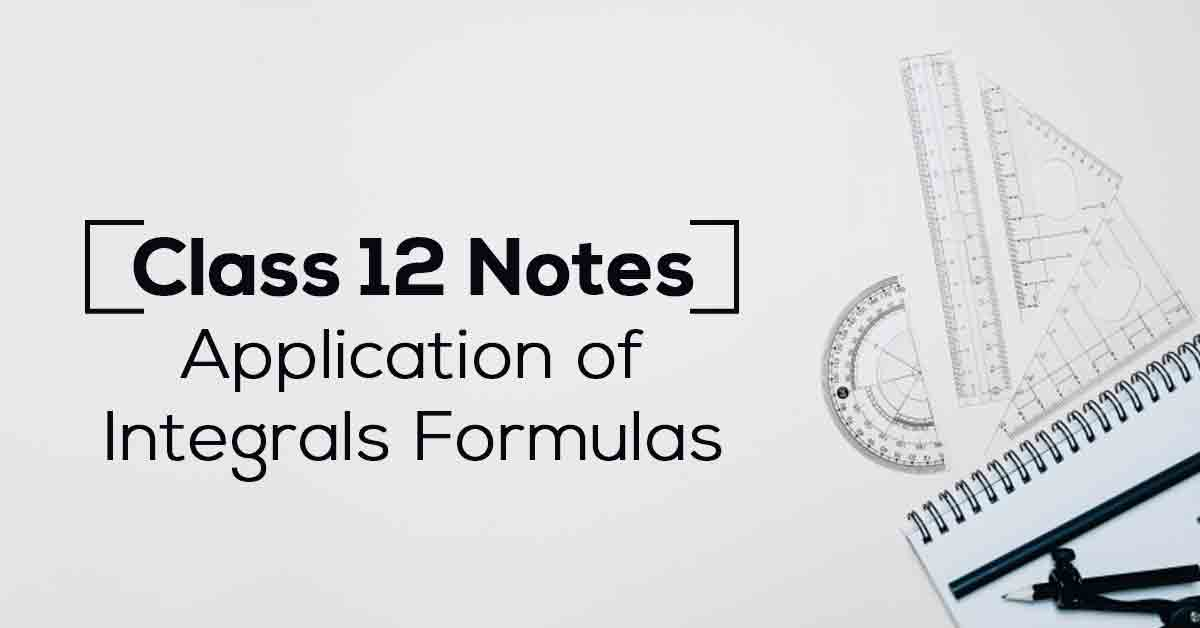Class 12th Math Application Of Integrals Formulas CBSE 2023

# Application of Integrals Class 12 Notes## Class 12 Maths Chapter 8 Application of Integrals Formulas – Pdf Download

Applications of the Indefinite Integral shows how to find displacement (from velocity) and velocity (from acceleration) using the indefinite integral. There are also some electronics applications in this section. Know More about these in Application of Integrals Class 12 Notes List.

The topics and sub-topics covered in Application of Integrals Class 12 Notes are:

8.1 Introduction

8.2 Area under Simple Curves

8.2.1 The area of the region bounded by a curve and a line

8.3 Area between Two Curves.

Download the FREE PDF of Application of Integrals Class 12 Notes and start your preparation with Vidyakul!